# Civil Engineering - Applied Mechanics

### Exercise :: Applied Mechanics - Section 3

16.

If v and ω are linear and angular velocities, the centripetal acceleration of a moving body along the circular path of radius r, will be

 A.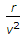B.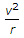C.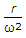D.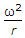E. rω.

Explanation:

No answer description available for this question. Let us discuss.

17.

If two forces of 3 kg and 4 kg act at right angles to each other, their resultant force will be equal to

 A. 7 kg B. 1 kg C. 5 kg D. 1/7 kg E. none of these.

Explanation:

No answer description available for this question. Let us discuss.

18.

The c.g. of the shaded area of the below figure from the x-axis is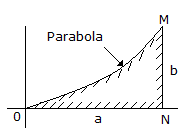A.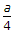B.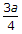C.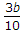D.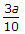E.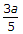Explanation:

No answer description available for this question. Let us discuss.

19.

Two shots fired simultaneously from the top and bottom of a vertical tower with elevations of 30° and 45° respectively strike a target simultaneously. If horizontal distance of the target from the tower is 1000 m, the height of the tower is

 A. 350 m B. 375 m C. 400 m D. 425 m.

Explanation:

No answer description available for this question. Let us discuss.

20.

For the given values of initial velocity of projection and angle of inclination of the plane, the maximum range for a projectile projected upwards will be obtained, if the angle of projection is

 A. α =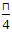-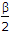B. α =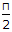+C. α =-D. α =-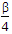E. α =-